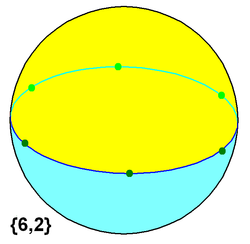# Dihedron

﻿
Dihedron
Set of regular n-gonal dihedronsExample hexagonal dihedron on a sphere
Type Regular polyhedron
or spherical tiling
Faces 2 n-gons
Edges n
Vertices n
Schläfli symbol {n,2}
Vertex configuration n2
Coxeter–Dynkin diagramWythoff symbol 2 | n 2
Symmetry group Dnh, [2,n], (*22n)
Dual polyhedron hosohedron

A dihedron is a type of polyhedron, made of two polygon faces which share the same set of edges. In three-dimensional Euclidean space, it is degenerate if its faces are flat, while in three-dimensional spherical space, a dihedron with flat faces can be thought of as a lens, an example of which is the fundamental domain of a lens space L(p,q).

Usually a regular dihedron is implied (two regular polygons) and this gives it a Schläfli symbol as {n, 2}.

The dual of a n-gonal dihedron is the n-gonal hosohedron, where n digon faces share two vertices.

## As a polyhedron

A dihedron can be considered a degenerate prism consisting of two (planar) n-sided polygons connected "back-to-back", so that the resulting object has no depth.

From a Wythoff construction on dihedral symmetry, a truncation operation on a regular {n,2} dihedron transforms it into a 4.4.n n-prism.

## As a tiling on a sphere

As a spherical tiling, a dihedron can exist as nondegenerate form, with two n-sided faces covering the sphere, each face being a hemisphere, and vertices around a great circle. (It is regular if the vertices are equally spaced.)

The regular polyhedron {2,2} is self-dual, and is both a hosohedron and a dihedron.

Regular dihedron examples: (spherical tilings)

## Ditopes

A regular ditope is an n-dimensional analogue of a dihedron, with Schläfli symbol {p,..q,r,2}. It has two facets, {p,...,q,r}, which share all ridges, {p,...,q} in common.